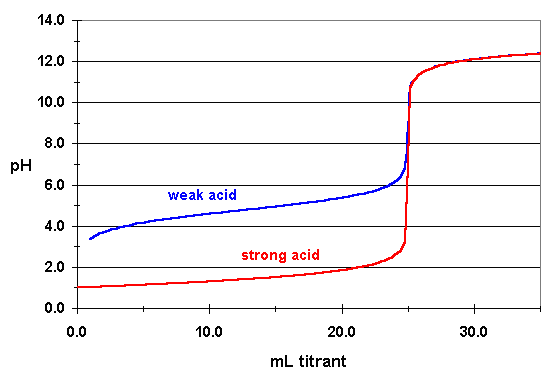# What is the difference between the titration of a strong acid with a strong base and that of the titration of a weak acid with a strong base?

Which of the following statements best characterizes the difference between the titration of a strong acid with a strong base and that of the titration of a weak acid with a strong base?

A) Both the strong acid and the strong base are completely dissociated in solution whereas the weak acid dissociation equilibrium constant is small enough that there is a measurable amount of the un-dissociated acid present in aqueous solution.

B) The equivalence point of a titration of a strong acid with a strong base may be observed with an indicator whereas the equivalence point of a titration of a weak acid with a strong base can only be observed with a pH meter.

C) The titration of a strong acid with a strong base is a fast reaction whereas the titration of a weak acid with a strong base is generally a very slow reaction.

This website seems to indicate that choice C is correct.

For B, all I know is that the pH at the equivalence point does not equal 7.00 (pH > 7.00) for the weak acid titration.

For A, I know the first part of that sentence is true. Strong acids and bases are characterized by completely dissociating whereas weak acids do not dissociate to the same extent. But is it true that there's a "measurable amount" of the un-dissociated acid present in aqueous solutions?

Let's look at a weak acid: acetic acid. It has a $K_a$ of $1.8\cdot 10^{-5}$. From this you can calculate how much it will dissociate in water. Let's say we have a concentration of $1~\mathrm{M}$:
This means, at equilibrium, $[\ce{CH3COOH}] = 1~\mathrm{M}- 4.233 \cdot 10^{-3} = 0.995~\mathrm{M}$. As you can see, by far the largest part is not dissociated!
B is not true, you can still observe the equivalence point. Have a look at the following picture, that shows the titration curve for weak / strong acid titrated with strong base. When choosing an indicator for colorimetric titration select one so that the pH jump at the equivalence point contains the interval $\mathrm{p}K_a\pm1$. Phenolphthalein has a $\mathrm{p}K_a \approx 9$, so to decide if it is a suitable indicator you would check if the pH jumps from 8 to 10 at the equivalence point. It does:C is not true either. If it was a very slow reaction, you could not titrate accurately, as you would have to wait a long time between every drop of $\ce{NaOH}$.